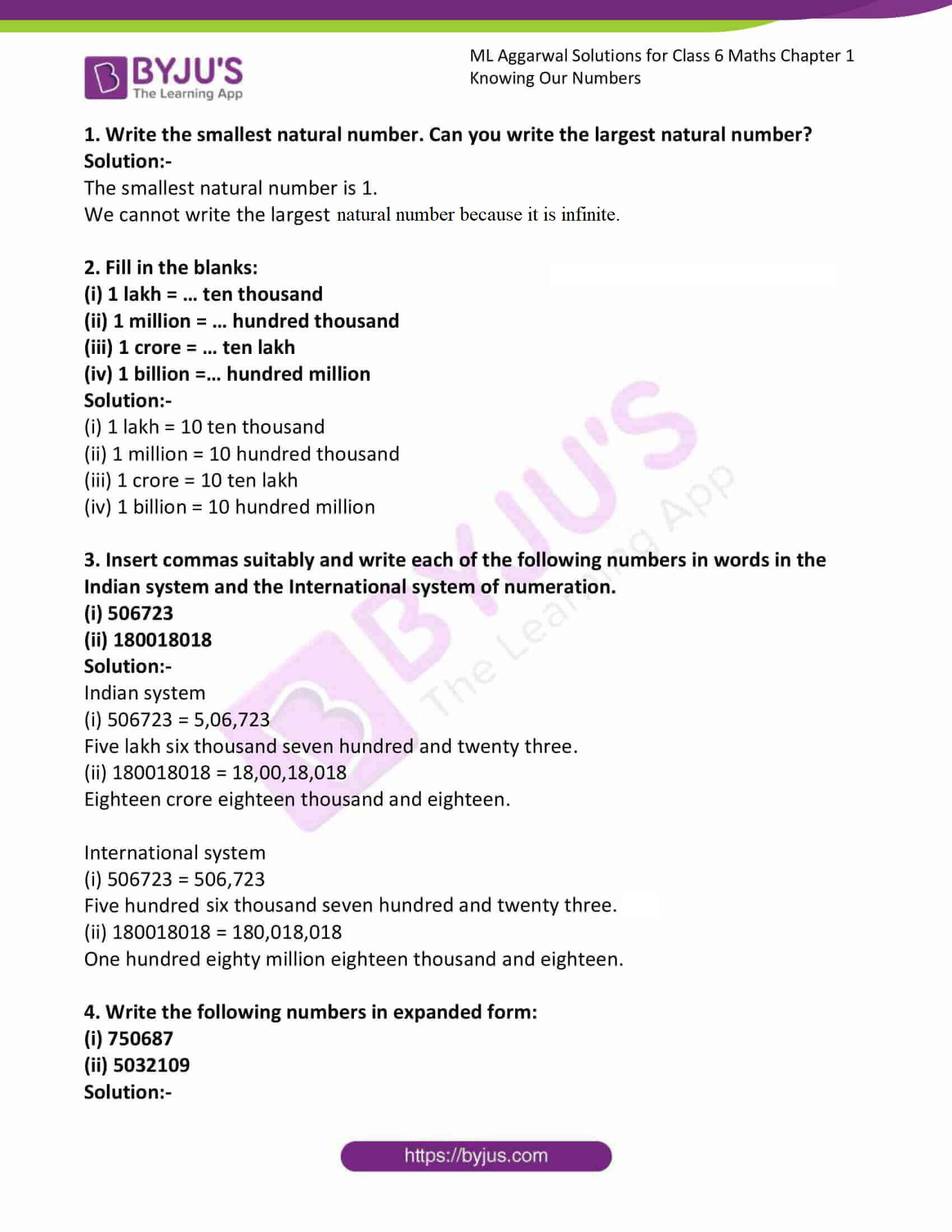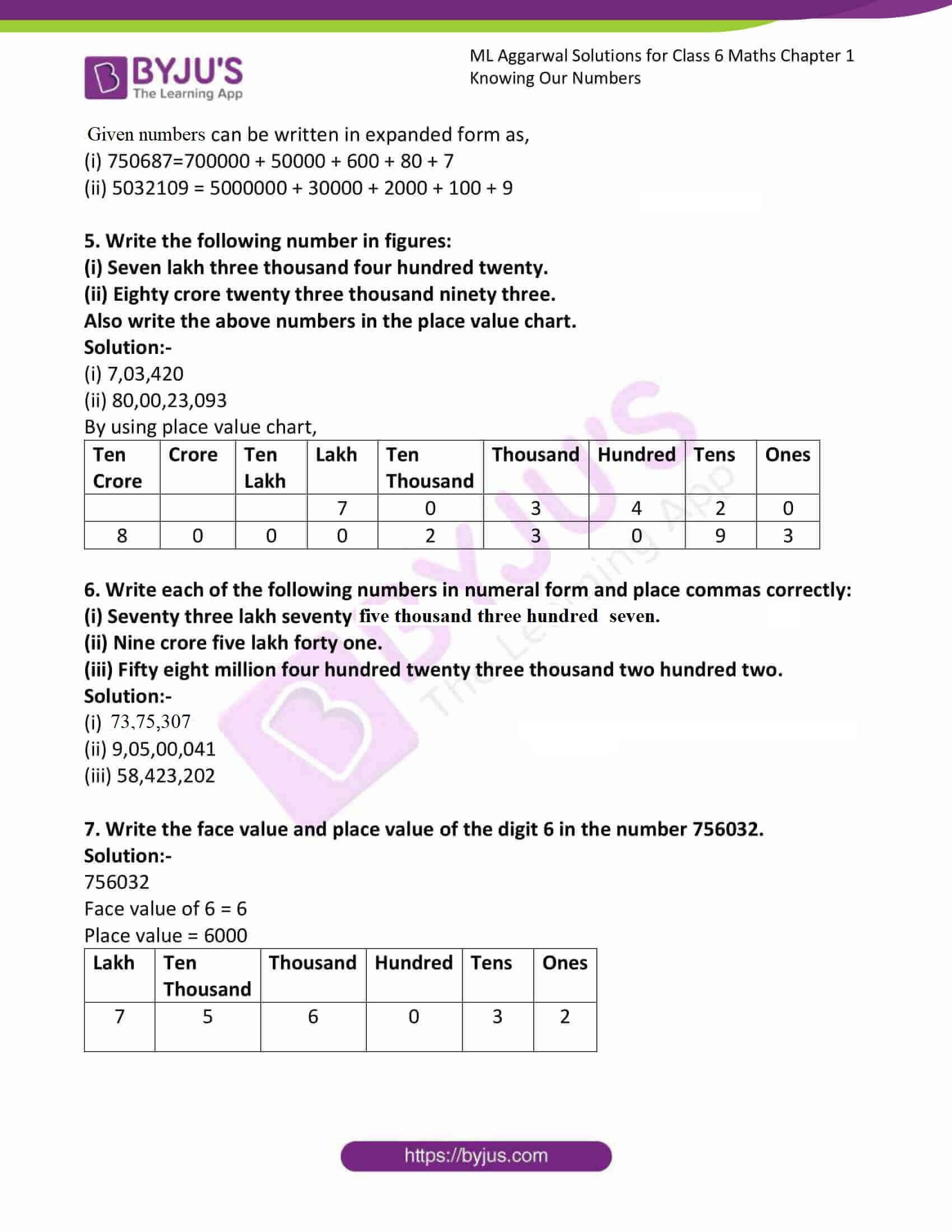# ML Aggarwal Solutions for Class 6 Maths Chapter 1 Knowing Our Numbers

ML Aggarwal Solutions for Class 6 Maths Chapter 1 Knowing Our Numbers are given here. When students feel stressed about searching for the most comprehensive and detailed solutions, ML Aggarwal Solutions is the best study material. We, at BYJU’S, have prepared step by step solutions. Students can either practice online or download these files and solve different types of questions related to this Chapter and achieve maximum marks in their examinations.

Chapter 1 – Knowing Our Numbers is based on representing or expressing numbers of a certain type is known as the number system. Now, let us have a look at some of the concepts discussed in the ML Aggarwal Solutions for Class 6 Maths Chapter 1, which include, reading and writing of large numbers, comparing large numbers, Indian System of Numeration, International System of Numeration.

## Download the PDF of ML Aggarwal Solutions for Class 6 Maths Chapter 1 Knowing Our Numbers### Access answers to ML Aggarwal Solutions for Class 6 Maths Chapter 1 Knowing Our Numbers

1. Write the smallest natural number. Can you write the largest natural number?

Solution:-

The smallest natural number is 1.

We cannot write the largest natural number because it is infinite.

2. Fill in the blanks:
(i) 1 lakh = … ten thousand
(ii) 1 million = … hundred thousand
(iii) 1 crore = … ten lakh
(iv) 1 billion =… hundred million

Solution:-

(i) 1 lakh = 10 ten thousand

(ii) 1 million = 10 hundred thousand

(iii) 1 crore = 10 ten lakh

(iv) 1 billion = 10 hundred million

3. Insert commas suitably and write each of the following numbers in words in the Indian system and the International system of numeration.
(i) 506723
(ii) 180018018
Solution:-

Indian system

(i) 506723 = 5,06,723

Five lakh six thousand seven hundred and twenty three.

(ii) 180018018 = 18,00,18,018

Eighteen crore eighteen thousand and eighteen.

International system

(i) 506723 = 506,723

Five hundred six thousand seven hundred and twenty three.

(ii) 180018018 = 180,018,018

One hundred eighty million eighteen thousand and eighteen.

4. Write the following numbers in expanded form:
(i) 750687
(ii) 5032109
Solution:-

Given numbers can be written in expanded form as,

(i) 750687=700000 + 50000 + 600 + 80 + 7

(ii) 5032109 = 5000000 + 30000 + 2000 + 100 + 9

5. Write the following number in figures:
(i) Seven lakh three thousand four hundred twenty.
(ii) Eighty crore twenty three thousand ninety three.
Also write the above numbers in the place value chart.
Solution:-

(i) 7,03,420

(ii) 80,00,23,093

By using place value chart,

 Ten Crore Crore Ten Lakh Lakh Ten Thousand Thousand Hundred Tens Ones 7 0 3 4 2 0 8 0 0 0 2 3 0 9 3

6. Write each of the following numbers in numeral form and place commas correctly:
(i) Seventy three lakh seventy five thousand three hundred seven.
(ii) Nine crore five lakh forty one.
(iii) Fifty eight million four hundred twenty three thousand two hundred two.
Solution:-

(i) 73,75,307

(ii) 9,05,00,041

(iii) 58,423,202

7. Write the face value and place value of the digit 6 in the number 756032.
Solution:-

756032

Face value of 6 = 6

Place value = 6000

 Lakh Ten Thousand Thousand Hundred Tens Ones 7 5 6 0 3 2